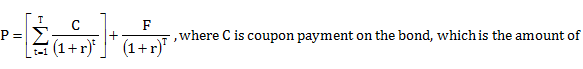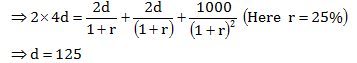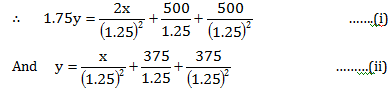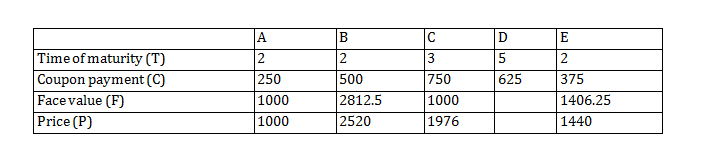Answer question nos. 1-3 based on the information given below:
Madhubala Devi, who works as a domestic help, received Rs. 2500 as Deepawali bonus from her employer. With that money she is contemplating purchase of one or more among 5 available government bonds – A, B, C, D and E.
To purchase a bond Madhubala Devi will have to pay the price of the bond. If she owns a bond she receives a stipulated amount of money every year (which is termed as the coupon payment) till the maturity of the bond. At the maturity of the bond she also receives the face value of the bond.
Price of a bond is given by:money the holder of the bond receives annually; F is the face value of the bond, which is the amount of money the holder of the bond receives when the bond matures (over and above the coupon payment for the year of maturity); T is the number of years in which the bond matures; r = 0.25, which means the market rate of interest is 25%.
Among the 5 bonds the bond A and another two bonds mature in 2 years, one of the bonds matures in 3 years, and the bond D matures in 5 years.
The coupon payments on bonds A, E, B, D and C are in arithmetic progression, such that the coupon payment on bond A is twice the common difference, and the coupon payment on bond B is half the price of bond A.
The face value of bond B is twice the face value of bond E, but the price of bond B is 75% more than the price of bond E. The price of bond C is more than Rs. 1800 and its face value is same as the price of bond A. The face value of bond A is Rs. 1000.
Bond D has the largest face value among the five bonds.

Question 1. The face value of bond E must be
A. Rs. 1406.25
B. Rs. 1686.25
C. Rs. 2250.50
D. Rs. 2812.50
E. Rs. 3372.50

Question 2. Madhubala Devi purchased one or more of the 5 available bonds from her bonus pay and spent the remainder. She made the purchase decision such that her return from the bonds is maximized. Her return from the bonds is
A. Rs. 3000.00
B. Rs. 3250.00
C. Rs. 3656.25
D. Rs. 3906.25
E. Rs. 4531.25

Question 3. The price of bond C must be
A. Rs. 1825
B. Rs. 1874
C. Rs. 1925
D. Rs. 1976
E. Rs. 2342

Solution: For question 1 to 3:

Let the coupon payment on bonds A, E, B, D and C be a – 2d,

a – d, a, a + d and a + 2d respectively.

Given that a – 2d = 2d ⇒ a = 4d.

Price of bond A = 2 × Price of bond B∴  Coupon payments of A = Rs. 250, B = Rs. 500, C = Rs. 750, D = Rs. 625 and E = Rs. 375.

The time of maturity of C can be either 2 or 3 years. Let it be 2 years.

∴   Price of bond C =∴   Time of maturity of bond C is 3 years and that of B and E is 2 years. Let the face value of bond E be Rs. x, then the face value of B is Rs. 2x. Let the prices of bond B and E be Rs. 1.75y and Rs. y respectively.Solving (i) and (ii), we get

x =1406.25 and y = 1440

Further analysis leads to the following table:Face value of Bond E is Rs. 1406.25.

Since Madhubala has an amount of Rs.2500 to invest, so she must have invested only in bonds A and E or only C. As she intends to maximize her return, she has to invest in bond A and E.

∴   Return = Face values of bond A and E + Coupon payment for both the bonds

= 1000 + 1406.25 + 2 × 250 + 2 × 375

= Rs. 3,656.25.